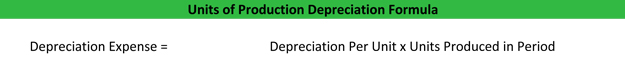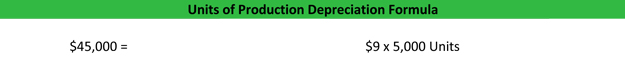# What are Units of Production Depreciation?

Definition: Units of production depreciation is a depreciation method that records depreciation expense for an asset based on the production levels of the asset over the useful life. In other words, the units of production depreciation method focuses on asset usage to book depreciation expense opposed to passage of time like more traditional deprecation methods.

## What Does Units of Production Depreciation Mean?

Since the units of production depreciation method uses the actual production levels of an asset to record deprecation expense, depreciation expenses can vary from year to year. Unlike straight line and double declining depreciation methods, units of production depreciation method is not based on a consistent percentage or table. because it is based on the usage of the asset. Some years the asset will be used more while some years the asset will be used less.

The units of production depreciation is calculated in a two step process. First the deprecation per unit is calculated using this equation.Once the depreciation per unit is calculated, the overall depreciation expense can be calculated.## Example

Let’s take a look at Western Machine Co. as an example. West bought a machine that cost \$200,000 on January 1 this year. The cost accountants at West believe the salvage value of the machine is \$20,000 and the machine will produce 20,000 units during its useful life. West calculates it depreciation per unit like this.This year West produced 5,000 units with its new machine. So at the end of the year, West will take its \$9 of depreciation per unit and multiply it by the 5,000 units produced to get the depreciation expense for the period.